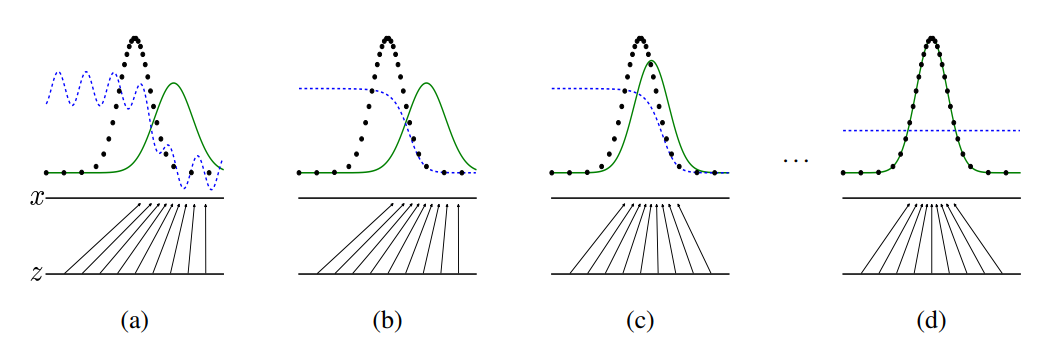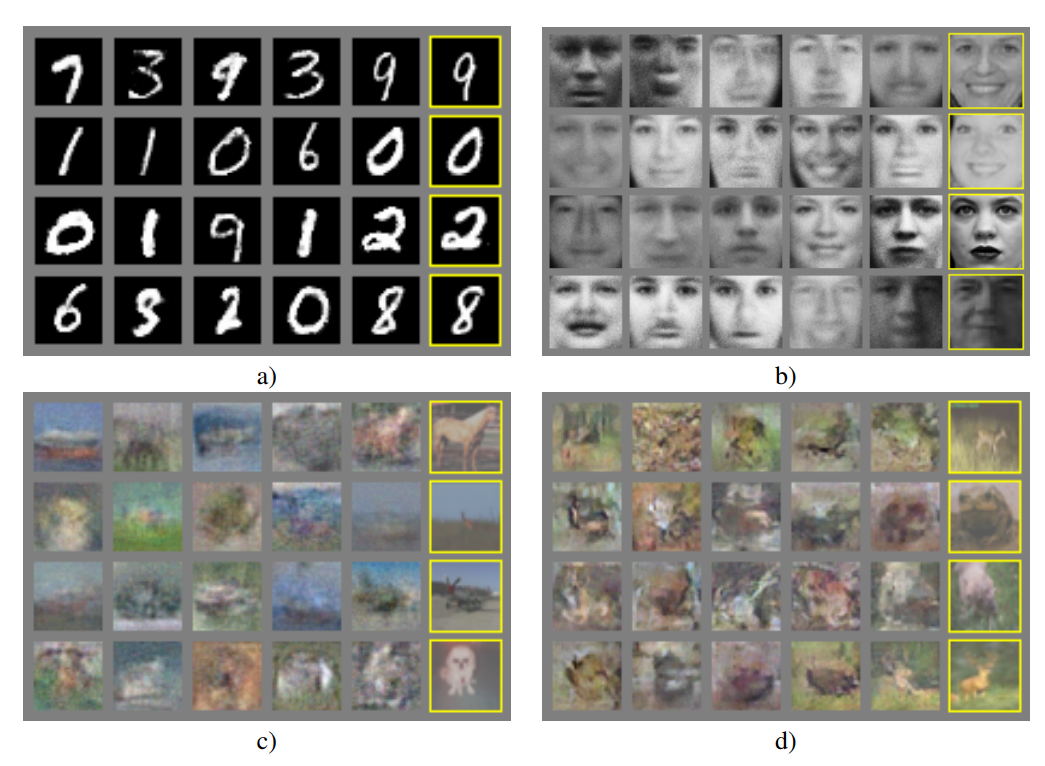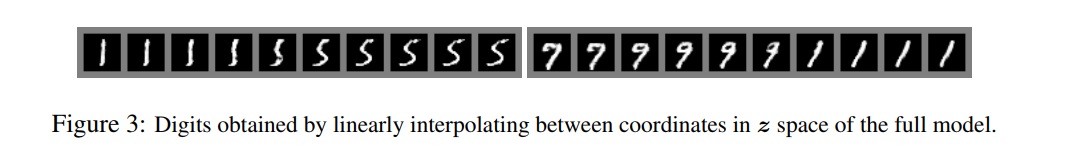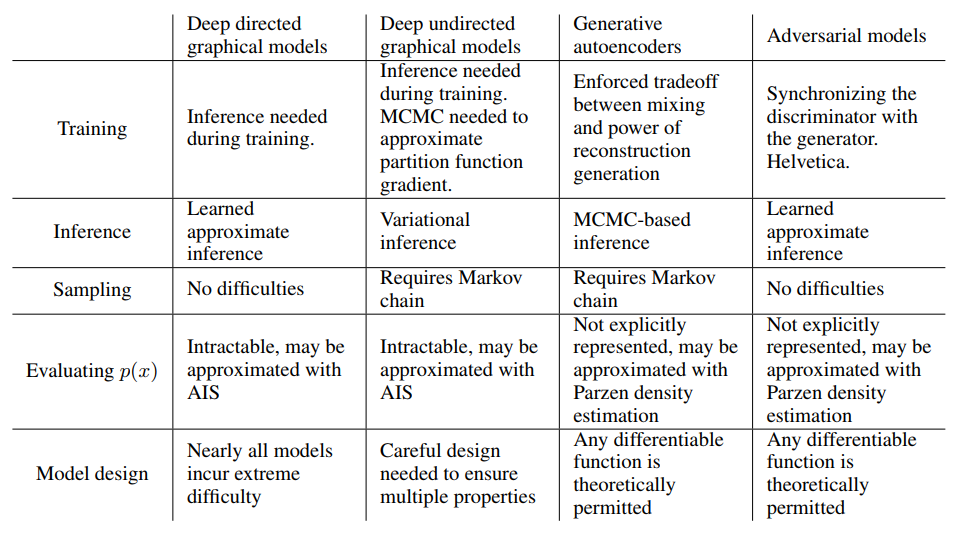# 2 相关工作

$\lim_{\sigma \to 0}\nabla_x E_{\epsilon \thicksim N{(0,\sigma^2I)}}f(x+\epsilon) = \nabla_xf(x)$

# 3 对抗网络

$\min\limits_G \max\limits_DV(D,G) = E_{x\thicksim{p_{data}(x)}}[\log D(x)] + E_{z\thicksim{p_z(z)}}[\log (1 - D(G(z)))] \tag{1}$（a）考虑靠近收敛的对抗对：$p_g$类似于$p_{data}$，D是部分准确的分类器。
（b）保持G不动，优化D，在算法D的内循环中训练以区分样本和数据，收敛到$D *(x)= \frac{p_{data}(x)} {p_{data}(x)+ p_g(x)}$，使得分类准确率最高。
（c）在每次更新G之后，D的梯度引导G（z）流向更可能被分类为数据的区域。即保持D不动，优化G，直到混淆程度最高。
（d）经过多次迭代后，如果G和D的性能足够，它们将达到两个都无法改善的点，因为$p_g = p_{data}$。判别器不能区分两个分布，即$D(x)= \frac{1}{2}$

——————————————————————————————————————————————————————————————————————————————————————————————

————————————————————————————————————————————————————————————————————————————————————————————————
for 训练迭代次数 do
for 优化 k 次 do
从$p_g(z)$选取的m个batch$\{z^{(1)},\cdot \cdot \cdot ,z^{(m)}\}$
从$p_{data}(x)$选取的m个batch$\{x^{(1)},\cdot \cdot \cdot ,x^{(m)}\}$
通过加上随机梯度来更新判别器
end for
从$p_g(z)$选取的m个batch$\{z^{(1)},\cdot \cdot \cdot ,z^{(m)}\}$
通过减去其随机梯度来更新生成器
end for

————————————————————————————————————————————————————————————————————————————————————————————————

# 4 理论成果

## 4.1 $p_g = p_{data}$具有全局最优性

### 命题1.

$\begin{array}{} V(G,D) & = \int_x p_{data}(x)\log(D(x))dx + \int_zp_z(z)\log(1-D(g(z)))dz \\ & = \int_xp_{data}(x)\log(D(x)) +p_g(x)\log(1-D(x))dx \tag{3} \end{array}$

$D$ 的训练目标可视为最大化估计条件概率$P(Y=y|x)$的对数似然。当$y=1$时，$x$来自$p-{data}$；当$y=0$时，$x$来自$p_g$。公式1的二元极小极大博弈可形式化为：
$\begin{array}{} C(G) & = max_DV(G,D) \\ &=E_{x \thicksim p_{data}}[\log D^*_G(x)] + E_{z \thicksim p_z(z)}[\log(1-D^*_G(G(x)))] \\ &=E_{x \thicksim p_{data}}[\log D^*_G(x)] + E_{x\thicksim p_g}[\log(1-D^*_G(x))] \\ &=E_{x \thicksim p_{data}}[\log \frac{p_{data}(x)}{p_{data}(x) +p_g(x)}] + E_{x\thicksim p_g}[\log \frac{p_g(x)}{p_{data}(x) +p_g(x)}] \tag{4} \end{array}$

$E_{x \thicksim p_{data}}[-\log 2]+E_{x \thicksim p_g}[-\log 2]=-\log 4$
$C(G)=V(G,D^*_G)$中减去上式，得：
$C(G)= -\log 4 + KL(p_{data}\mid\mid \frac{p_{data}+p_g}{2}) + KL(p_g\mid\mid \frac{p_{data}+p_g}{2})\tag{5}$

$C(G) = -\log 4 + 2\cdot JSD(P_{data}\mid\mid p_g)\tag{6}$

## 4.2 算法1的收敛性

### 命题2.

$E_{x \thicksim p_{data}}[\log D^*_G(x)] + E_{x \thicksim p_g}[\log (1-D^*_G(x))]$

,且对于每一个$\alpha$$f_\alpha(x)$关于$x$时凸的，那么，如果$\beta=arg sup_{\alpha\in A}f_ \alpha(x)$,则$∂f_{\beta}(x) \in ∂f$。等价于黑顶对应的G和最优判别D，梯度下降更新$p_g$。在定理1 中证明$sup_D U(p_g,D)$关于$p_g$是凸的且具有唯一的全局最优解，因此，$p_g$更新足够小时，$p_g$收敛到$p_x$，证毕。

# 5 实验a）MNIST
b）TFD
c）CIFAR-10（完全连接模型）
d）CIFAR-10（卷积鉴别器和“反卷积”发生器）Model MNIST TFD
DBN 138±2 1909±66
Stacked CAE 121±1.6 2110±50
Deep GSN 214±1.1 1890±29

#6 优点和缺点#7 结论和拓展

1. 添加$c$$G$$D$的输入，可获得条件的生成模型$p(x|c)$
2. 给定$x$，为预测$z$，训练任意的网络可学习近似推理。类似于 wake-sleep 算法训练出的推理网络，但训练推理网络时可能要用到训练完成后的固定的生成网络。
3. 来近似建模所有的条件概率$P(x_S|x_S)$，其中，$S$为通过训练共享参数的条件模型簇的$x$的索引。本质上，对抗的网络可用于随机扩展 MP-DBM。
4. 半监督学习：当标签数据有限时，判别网络或推理网络的特征不会提高分类器效果。
5. 效率改善：为协调$G$$D$设计更好的方法，或训练期间确定更好的分布来采样$z$，从而加速训练。

# 补充

OpenAI Ian Goodfellow的Quora问答

## 与其他生成式模型相比较，生成式对抗网络有以下四个优势：

1、根据实际的结果，它们看上去可以比其它模型产生了更好的样本（图像更锐利、清晰）。
2、生成对抗式网络框架能训练任何一种生成器网络（理论上-实践中，用 REINFORCE 来训练带有离散输出的生成网络非常困难）。大部分其他的框架需要该生成器网络有一些特定的函数形式，比如输出层是高斯的。重要的是所有其他的框架需要生成器网络遍布非零质量（non-zero mass）。生成对抗式网络能学习可以仅在与数据接近的细流形（thin manifold）上生成点。
3、不需要设计遵循任何种类的因式分解的模型，任何生成器网络和任何鉴别器都会有用。
4、无需利用马尔科夫链反复采样，无需在学习过程中进行推断（Inference），回避了近似计算棘手的概率的难题。

## GAN目前存在的主要问题：

### 2、难以训练：崩溃问题（collapse problem）

GAN模型被定义为极小极大问题，没有损失函数，在训练过程中很难区分是否正在取得进展。GAN的学习过程可能发生崩溃问题（collapse problem），生成器开始退化，总是生成同样的样本点，无法继续学习。当生成模型崩溃时，判别模型也会对相似的样本点指向相似的方向，训练无法继续。

【Improved Techniques for Training GANs】